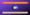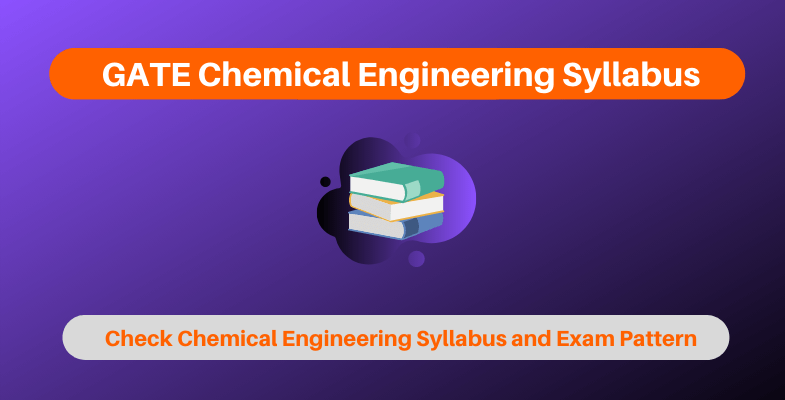#GATE Chemical Engineering Syllabus: To prepare Chemical Engineering Exam, you must know the latest GATE Chemical Engineering Syllabus and marking scheme. The latest GATE Syllabus lets you know the important chapters and concepts to be covered in all subjects.

With the clear concepts in every topic of Chemical Engineering, you can crack the Gate exam.

Here we are providing you the complete guide on GATE Chemical Engineering Syllabus 2020 and Marking Scheme.

## GATE Chemical Engineering Syllabus 2021

Chemical Engineering is one of the popular engineering streams among students. The branch uses the basic principles of Chemistry, Physics, Biology, Maths, etc to transform energy and material into useful products. Through GATE candidates can appear for Chemical Engineering paper and take admission in M.Tech courses offered at prestigious institutes of India.

GATE Authority publishes the latest syllabus for all branches in its official Website. With the latest Chemical Engineering Syllabus, you can create a solid study plan and score a better mark in all GATE exam.

### Topics from Chemical Engineering

• Section 1 – Process Calculations and Thermodynamics :
• Steady and unsteady state mass and energy balances including multiphase, multi-component, reacting and non-reacting systems. Use of tie components; recycle, bypass and purge calculations; Gibbs phase rule and degree of freedom analysis.
• First and Second laws of thermodynamics. Applications of first law to close and open systems. Second law and Entropy. Thermodynamic properties of pure substances: Equation of State and residual properties, properties of mixtures: partial molar properties, fugacity, excess properties and activity coefficients; phase equilibria: predicting VLE of systems; chemical reaction equilibrium.
• Section 2 – Fluid Mechanics and Mechanical Operations
• Fluid statics, Newtonian and non-Newtonian fluids, shell-balances including differential form of Bernoulli equation and energy balance, Macroscopic friction factors, dimensional analysis and similitude, flow through pipeline systems, flow meters, pumps and compressors, elementary boundary layer theory, flow past immersed bodies including packed and fluidized beds, Turbulent flow: fluctuating velocity, universal velocity profile and pressure drop.
• Particle size and shape, particle size distribution, size reduction and classification of solid particles; free and hindered settling; centrifuge and cyclones; thickening and classification, filtration, agitation and mixing; conveying of solids.
• Section 3 – Heat Transfer
• Steady and unsteady heat conduction, convection and radiation, thermal boundary layer and heat transfer coefficients, boiling, condensation and evaporation; types of heat exchangers and evaporators and their process calculations. Design of double pipe, shell and tube heat exchangers, and single and multiple effect evaporators.
• Section 4 – Mass Transfer
• Fick’s laws, molecular diffusion in fluids, mass transfer coefficients, film, penetration and surface renewal theories; momentum, heat and mass transfer analogies; stage-wise and continuous contacting and stage efficiencies; HTU & NTU concepts; design and operation of equipment for distillation, absorption, leaching, liquid-liquid extraction, drying, humidification, dehumidification and adsorption.
• Section 5 – Chemical Reaction Engineering
• Theories of reaction rates; kinetics of homogeneous reactions, interpretation of kinetic data, single and multiple reactions in ideal reactors, non-ideal reactors; residence time distribution, single parameter model; non-isothermal reactors; kinetics of heterogeneous catalytic reactions; diffusion effects in catalysis.
• Section 5 – Instrumentation and Process Control
• Measurement of process variables; sensors, transducers and their dynamics, process modeling and linearization, transfer functions and dynamic responses of various systems, systems with inverse response, process reaction curve, controller modes (P, PI, and PID); control valves; analysis of closed-loop systems including stability, frequency response, controller tuning, cascade and feed forward control.
• Section 8 – Plant Design and Economics
• Principles of process economics and cost estimation including depreciation and total annualized cost, cost indices, rate of return, payback period, discounted cash flow, optimization in process design and sizing of chemical engineering equipment such as compressors, heat exchangers, multistage contactors.
• Section 9 – Chemical Technology
• Inorganic chemical industries (sulfuric acid, phosphoric acid, chlor-alkali industry), fertilizers (Ammonia, Urea, SSP and TSP); natural products industries (Pulp and Paper, Sugar, Oil, and Fats); petroleum refining and petrochemicals; polymerization industries (polyethylene, polypropylene, PVC and polyester synthetic fibers).

### Topics from Engineering Mathematics

• Linear Algebra: Matrix algebra, Systems of linear equations, Eigenvalues and Eigenvectors.
• Calculus: Functions of single variable, Limit, continuity and differentiability, Taylor series, Mean value theorem, Evaluation of definite and improper integrals, Partial derivatives, Total derivative, Maxima and minima, Gradient, Divergence and Curl, Vector identities, Directional derivatives, Line, Surface and Volume integrals, Stokes, Gauss and Green’s theorems.
• Differential equations: First order equations (linear and nonlinear), Higher order linear differential equations with constant coefficients, Cauchy’s and Euler’s equations, Initial and boundary value problems, Laplace transforms, Solutions of one-dimensional heat and wave equations and Laplace equation.
• Complex variables: Complex number, polar form of complex numbers, triangle Inequality.
• Probability and Statistics: Definitions of probability and sampling theorems, Conditional probability, Mean, median, mode and standard deviation, Random variables, Poisson, Normal and Binomial distributions, Linear regression analysis.
• Numerical Methods: Numerical solutions of linear and non-linear algebraic equations. Integration by trapezoidal and Simpson’s rule. Single and multi-step methods for numerical solution of differential equations.